International Journal of Mechanical Engineering and Applications
Volume 3, Issue 1-3, February 2015, Pages: 69-75

Optimizing repair interval of vehicle based on reliability

Vo Trong Cang1, 2

1Faculty of Transportation Engineering, Ho Chi Minh City University of Technology, Ho Chi Minh City, Vietnam

2Hoanmy Engineering Co. Ltd. Ho Chi Minh City, VietnamVo Trong Cang. Optimizing Repair Interval of Vehicle Based on Reliability. International Journal of Mechanical Engineering and Applications. Special Issue: Transportation Engineering Technology. Vol. 3, No. 1-3, 2015, pp. 69-75. doi: 10.11648/j.ijmea.s.2015030103.21

Abstract: The maintenance system should be built taking into account the specific exploiting conditions, that is, to take into account not only the running distance between repairs, but the volume of the planned maintenance was made (both preventive and corrective) and their intervals. The paper presents principles and algorithms to optimize the meantime between repairs for the components of vehicles at a given level of reliability parameters. This algorithm can be applied to the worn parts of vehicles through the attrition survey in the specific exploiting conditions and calculating the reliability indexes respectively.

Keywords: Optimization, Mean Time to Repair, Vehicle Maintenance, Reliability

1. The Failure Flow Parameter During the Working Time

The failure flow parameter changes during the time of work, as shown in Fig 1.1 . This process can be divided into three stages:

Stage I - running clearance:

After the start of use or after the repair of equipments, their failure flow parameter increases sharply, and then will gradually decrease to a level determined. This characteristic of the relationship ω(l) is due to the quality of manufactured parts and details, the existence of hiden defects, the violations of technology when manufacturing or repairing.

Stage II-normal use:

This stage is characterized by the unchanged failure flow parameter. In this stage appear the extraordinary damages, which mainly occur due to random causes and are distributed relatively evenly during the working time. Failure flow parameter in this stage is determined by the characteristics and structural perfection of equipments, as well as the conditions and exploitation mode of equipments.

Stage III - increased wear or aging:

The basic features of this stage are the failure flow parameter increased that are caused by several factors such as:

- The abrasion and aging of the parts and details lead to an increase in the probability that the pulse load exceeds the limit of their strength;

- The increase in the gap within the assemblied elements leads to the increase in the vibration, shock and momentum;

-The occurrence of the excessive within the moveable joints leads to the decrease in their mechanical strength;

-The decrease in electrical resistance of electro-insulating materials.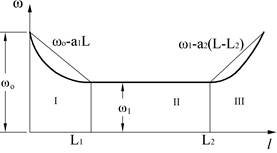Figure 1.1. The change of the failure flow parameter during the working time

To prevent the failure of parts and weared details we set the preventive-planned repairing system, in which we undertook to restore or replace the details and components with the technical specs close to the critical values .

On the other hand, the establishment of the planned repair, which is before the stage of wear and aging with the increase in damages, is meaningless.

For example, the implementation of the planned repair at the time of L1 (Fig. 1.2) is not only beneficial, but harmful. At this time the number of unscheduled repairs increases due to the occurrence of the damages of running clearance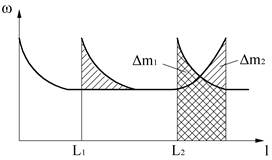Figure 1.2. The change of the failure flow parameter in difference distances running to repair

Here, the scheduled repair is logical if it is made at the time of any L2, where the number of damages Δm2 is greater than the number of damages of running clearance Δm1 (characterized by the growth of the failure flow parameter).

Should determine a reasonable time to conduct the planned repair, in which the total cost for the conduct of repair (planned and unplanned) is minimal .

If at the planned repair we carry out the fully restore of the parts, i.e. the function w(l) will be restored completely after repairs as had earlier, then during the long enough working time of the means, the relationship w(l) will have the form as shown in Fig 1.3. Here, L, 2L, 3L ... correspond to the times of the planned repairs.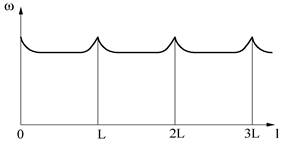Figure 1.3. The change of the failure flow parameter within the intervals between repairs.

Because the relationships w(l) in the intervals [0,L], [L,2L], [2L,3L] ... are completely the same, so just consider an interval [0, L] is sufficient.

The number of unscheduled repairs in the interval [0, L] is equivalent to the amount of damages:

H(l) =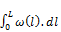,                       (1.1)

Thus the average number of damages within the long enough working time l will be:NDX = H(l) =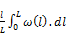,              (1.2)

The number of times to repair NKH planned in the interval [0, l] is:

NKH =,                                     (1.3)

Let CKH is the average cost for one planned repair, and CDX is the average cost for one unscheduled repair considering the loss of the stopping of the means, then the total cost for the conduct of both planned and unplanned (unscheduled) repairs in the interval [0, l] is:

C = CDX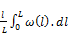+ CKH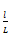,               (1.4)

Upon dividing this expression for l, we obtain the average total cost for the repair:

q(L)=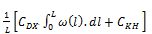,             (1.5)

The quantity q(L) is the optimum target of the interval between repairs of the elements of the means, and it is received from their failure information. To calculate the optimum target q(L) by Eq. 1.5 we need to know the relationship of the failure flow parameter ω with working time l. In general form, this relationship can be approximated in high accuracy with the polynomial of n-th degree:

w(l ) =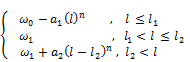To simplify, this relationship can be approximated with the piecewise-linear method. This approximation can satisfy in the real situation [1,2].

We assume that (0, l1) and (l2, L) are small enough, the wear will intensively increase in the stages I and III, the function will be:

w(l) =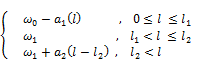In this case, the compolsary repair should be planned at the appropriate period uppon the severe wear - when the damage is increased rapidly, i.e. L > l2. Then, the integral in Eq. 1.4 are splitted into three: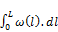=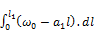+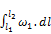+

+Upon transforming with a1 =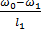we have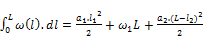(1.6)

Upon replacing (1.6) into (1.5), we obtain

q (L) =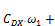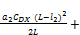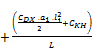(1.7)

Indeed, the function q(L) is the polynomial of 2nd degree with one minimum point (see Fig. 1.4).

The second termis positive increased with the distance between the repairs L, while the third term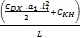is negative; the term CDX1 is constant within the full range of variable of the interval between the repairs and it does not affect the horizontal position L0 of the minimum point, but affects the release of q(L0).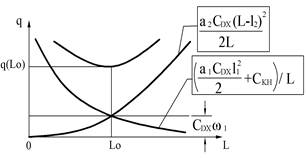Figure 1.4. The Relationship between the total units cost with the interval between repairs

To determine the optimal interval between the repairs we take the derivative of q(L) and give it equal to 0: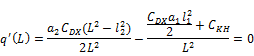Of which:

L0 =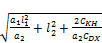(1.8)

From the expression (1.8) in particular, we found that planned repair should be conducted in the stage of increasing damages, as L0> l2.

Also, when there is no damage specific to the wear and running clearance, i.e. when a1→0, a2→0, ω(l)→ω1= const, the optimal interval between repairs L0→∞, i.e. no need to carry out planned repairs, because it does not prevent the unexpected failures.

The unexpected failures affect the absolute value of the total unit cost for both the planned and unplanned (unscheduled) repairs.

This is seen from expression (1.5), because of which the CDXω1, where ω1 is the value of failure flow parameter during the stage of normal use, in which the unexpected failures of the considered components are overwhelming.

However, the abscissa of the minimum point of the total unit cost does not depend on the value of ω1, as shown in expression (1.8), but is determined mainly by the speed of the changes of the failure flow parameter within the stage of running clearance (a1) and within the stage of the increased wear or aging (a2).

The time for running clearance l1 depends greatly on the type of previous repair, so there will not any information about it, while the structure of the repairing cycle is being determined.

In addition, the period of the running clearance l1 represents only a fraction of normal use period [l1, l2], so we can ignore the first term in the expression (1.8).

Thus, the optimal interval between the repairs is determined primarily by the time of the normal use period [l1, l2] and depends on the relationship (ratio) of the cost of the planned repair CKH and the cost of the unplanned repair (unscheduled) CDX , as well as the speed of the increase in the failure flow parameter in the stage of increased wear or aging a2, i.e. the intensity of these processes.

This intensity allows forecast the life of the components which are under wear and aging. Such the relationship of the failure flow parameter with the working time has been shown in several studies (e.g. [1,2,6]).

Featuring change of such failure flow parameter, its function can be expressed as straight lines:(1.9)

The expression of the function ω(l) consists of eight coefficients (a1, a2, a3, l1, l2, b1, b2, b3) and should determined by the ready empirical relationships ω*(l), i.e. the graph of experimental failure flow parameter. However, there are several redundant factors, so due to the conditions of continuity of the function ω(l), we have the boundary equations as follows:

ω 1(l1) = ω 2(l1)   and    ω 2(l2) = ω 3(l2)

From this, we have:

b2 = a1l1 + b1 ;

b3 = a2 (l2 - l1) + a1 l1 + b1 .

Finally, the function of the failure flow parameter has the form as:

ω1(l) = a1 l + b1   ,                     0 ≤ l ≤ l1

ω2(l) = a2(l - l1) + a1 l1 + b1 ,                 l1 < ll2

ω3(l) = a3(l - l2) + a2(l2 - l1) + a1l1+ b1 ,                 l2 <  l ,  (1.10)

Here, the expression of ω(l) consists only of six constants: a1, a2, a3, b1, l1, l2, but to define the problem we have to approximate the empirical chart of the experimental failure flow parameter ω*(l) with the theoretical ω(l).

The approximation, (i.e. replacing the empirical function ω*(l) with the theoretical ω(l),) in this case will give more accurate results, if the amount of damages, calculated in accordance ω*(l) and ω(l) will have a minimum deviation, i.e. considering the requirements of the least squares method.

The objective function for the approximation of the failure flow parameter would be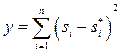(1.11)

of which:

Si and Si*- the corresponding numbers of theoretic and empirical damages counted in the i-th interval of working time;

n - number of working time interval (i.e. the steps of the diagram).

The number of empirical damages Si* will determined by the rectangular area of the i-th interval of the ω*(l) chart:

Si*= ω i Δi*l                           (1.12)

Using piecewise-linear approximation the theoretic amount of damages Si is determined by the trapezoidal area of li–1; ω(li - l); li , i.e.

Si = ω(Xi) Δl  ,                           (1.13)

of which:

ω(Xi) - theoretical value of the function ω(l) at the midpoint (median) of the i-th interval;

Δ l - the average line of the trapezoid.

Replacing (1.12) and (1.13) into (1.11), we get: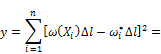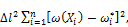(1.14)

Because that Δl2 is constant, the optimal condition of Eq. 1.14 is equivalent to the minimum objective function: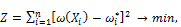(1.15)

Whereas, the graph approximation of the failure flow parameter is changed into the piecewise-linear approximation of the empirical function ω*(l), which is given by n points coordinated of (xi; ω*i), where xi is the middle point of the i-th interval of the ω*(l) chart;

ωi* - is the value of the failure flow parameter in the i-th interval

Replacing with (1.11), we can write the (1.15) as following: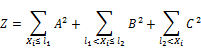where: A, B, C - the deviation of the failure flow parameters’ function, compared with the corresponding empirical values, on the changing intervals of  working time respectively:

A = a1 xi + b1 - ω1*;

B = a2 (xi - l1) + a1l1 + b1 - ωi*;

C = a3 (xi - l2) + a2(l2 - l1) + a1l1 + b1 - ωi*

The objective function (1.15) depends on the parameters a1, a2, a3, l1, l2, b1, but note that l1 and l2 coincide with the boundaries of the range of the ω*(l) graph, then their defining region is limited by the l1, l2, ..., ln .

So to degrade the system of variables, we should fix the values of l1 and l2 and determine the local minimal of the objective function z. We should repeat the solution with different combinations of l1 and l2, etc. … then chose from all of the answers the solution that ensures the minimum value of the objective function. Thus, while fixed l1 and l2, the four variable function z=(a1, a2, a3, b1)  is minimal.

2. Determine the Optimal Working Time

To treat the integral function of the failure flow parameter within Eq. 1.5, we insert one, two or three integral components depending on the type of the function w(l) within [0, L]: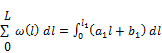+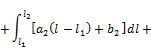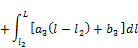Uppon calculating the integral we obtain (2.1):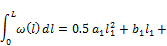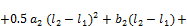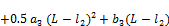(2.1)

Replacing expressions of the coefficients b2 and b3 into (1.6), then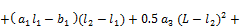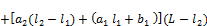(2.2)

We replace (2.2) into (1.5), then take the derivative by L and give it equal to 0, we get the expression, which determine the optimal interval between the repairs:Lo =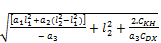,            (2.3)

Analysis of the expression (2.3) shows that the optimal interval between the repairs depends significantly on the relationship of the costs for carrying out repairs planned CKH and unplanned CDX.

The planned repair cost includes the cost for materials or spare parts C1, the cost for wages C2 and the cost for stop of the means C3, i.e.:   CKH=C1+C2+C3.

The cost for unplanned repair, in addition to the stated quantities C1, C2 and C3, also includes the cost C4, which is caused by damage of the vehicle during operation, i.e.:

CDX=C1+C2+C3+C4.

Thus, CDX ≥ CKH , and CDX = CKH only happens to the elements, whose damages do not cause to the vehicle’s loss during operation.

So far, still no formal studies on the determination of damage caused by the stop of the means halfway due to their failures. So if there were, they would not fully reflect the damages caused due to failures [5, 6, 7].

So, although the companies of transportation have information about the time to stop the means due to failures, it did not allow assessment of the C4 within the unplanned repairing cost. Consequently, it can not compute the absolute value of the average total cost of the repairs.

However, using the relative values of CDX and CKH, we can determine the optimal interval between the repairs L0, corresponding to the minimum value of the average total unit costs q(L0).

The ratio K of repair costs for planning and unplanned is: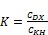(2.4)

Because CDX≥CKH   then  K ≥1.

Perform CDX by K and CKH , then replace it into Eq. 1.5, we get:

q(L)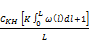,               (2.5)

On the physical sense, the quantity, located in the numerator of the fraction (2.5), is the total cost of both types of repairs. So, the expression in parentheses is the number of total converted repairs, i.e. the quantity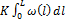turns the total number of unplanned repairsinto the cost-equivalent amount of planned repairs.

The relationship S(L) is called the number of converted unit repairs during one unit of working time:

S(L) =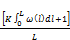,                    (2.6)

From the above theoretical basis, we set the algorithm for the approximation of the failure flow parameter, as shown in Fig 2.1.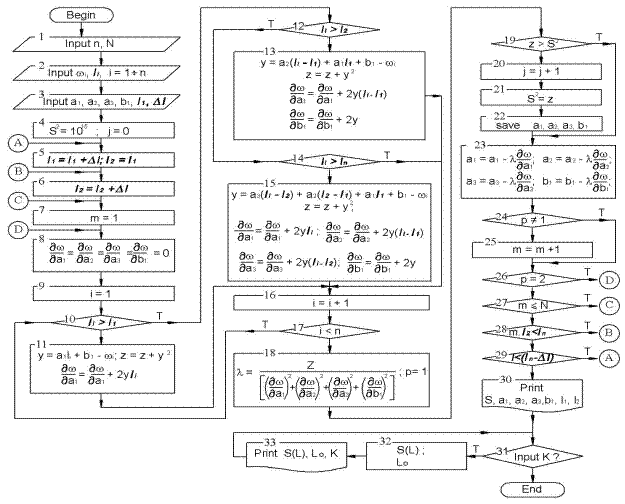Figure 2.1. Algorithm for piecewise-linear aproximation

Uppon enterring the number of values n – the amount of experimental data of the failure flow parameter (block 1), then assigning N - the number of repetitions of the coefficients until they were stable and the value of the objective function does not fall again .

Then, enter the experimental values of the failure flow parameter w*i and the initial values of the coefficients a1.1, a2.1, a3.1, b1.1 of the failure flow parameter (in the blocks 2 and 3).

Given (in the block 4) the large initial values of the objective function, which later will be compared with the squared deviation of the theoretical from the experimental values of the failure flow parameter after the first calculation (in block 19).

Given (in the blocks 5 and 6) the initial boundary values l1 and l2 of the failure flow parameter and the initial calculating values; the variables used for the accumulation of totals is given by 0 (in the block 8).

Then we perform one cycle of counting to determine a1, a2, a3, b1 (through the blocks 9 to 17) and the value of the coefficient λ (block 18) .

We remember the coefficients corresponding to the minimum value of the objective function z (through the blocks 19 to 22), and then calculate the new values of the coefficients a1, a2, a3, b1 (block 23).

The calculations by the "steepest descent method" should repeat (the blocks 24-27) until the total squared errors z will not decrease further during the N cycles.

Conduct one cycle of counting for all possible values of l1 and l2 (in the blocks 28 and 29). We send the calculated values of a1, a2, a3, b1 and the working times l1, l2 to the printer (block 30).

To calculate the value of the function S(L) by the coefficient K, we enter the value of K in the block 31; calculate the value of S(L) and L0, in the block 32, and then print the L0. The loop will proceed with all the input values of K. Finally, the program plots the graphs of S(L) and ends in the block 34.

Based on the theory mentioned, we can build the program for calculation of the function S(L), which denotes the relationship of the number of total converted unit repairs during the working time S(L) with the different values of K - the ratio of the cost of unplanned repairs to the cost of planned repairs. Corresponding each value of K we should find the optimal values of the repairing cycle and plot the graphs.

3. Conclusion

The programs, which calculating the relationships between the failure flow parameter w(l) and the number of the total converted units repairs with the working time S(L) will be useful tools for the determination of the optimal repairing cycle of the parts and components of the means with regard the unexpected failures and the correlation between the costs of planned and unplanned repairs respectively.

Following the studies [2-4] and [8-10] on the optimal repairing system based on the reliability, this paper is a part of the R&D project on the optimizing repairing cycle based on the reliability  and is funded by Vietnam National University Ho Chi Minh City (VNU-HCM) under grant number C2014-20-04 .

Acknowledgement

The author would like to express the gratitude to Prof. Dr. Do Duc Tuan with the Faculty of Mechanical Engineering, University of Transport and Communication, Ha Noi, by his valuable contribution to the article content.

References

1. Do Duc Tuan. Evaluation of wear, durability and reliability of details  and constructions of diesel locomotives. Pub of Transport, Hanoi, 2005
2. Do Duc Tuan. Optimizing fundamentals for repair period of details and units in locomotives with consideration of non-parameter failures and repair cost in and out of plan. Transport and Communications Science Journal. No. 16, pp. 125-136, Dec. 2006
3. Do Duc Tuan, Vo Trong Cang. Optimizing repair periods for components in locomotives at pre-set parameter reliability. Transport and Communications Science Journal. No. 17, pp. 134-142, Apr. 2007
4. Do Duc Tuan. Fundamentals for determination of optimal structure of locomotives repair cycle based on repair cost and gamma percent life cycle of elements. Transport and Communications Science Journal. No 21, pp. 134-142, Mar. 2008
5. Galkin V.G., Paramzin V.P., Tretverov V.A. Reliability of Locomotives. Moscow, Transport, 1981
6. Gorski A.V, Vorobiov A.A. Optimization of Locomotives’ Repair System/. Moscow, Transport, 1994
7. Puzankov A.D. Reliability Design of Locomotives. Moscow, MIIT., 1999
8. Vo Trong Cang. Optimizing locomotive repair cycle based on reliability. R&D Proj. Report T-KTGT-2010-06. HCM City University of Technology, 2011 (un published)
9. Vo Trong Cang. Optimizing repair interval of vehicle at a given level of reliability parameters. Science & Technology Development, Vol 17, No.K7- 2014, pp 35-44
10. Vo Trong Cang, Do Duc Tuan. Determination of the repair cycle optimized in consideration with repair costs and pre-set reliability parameters of the means of transport. J.Transportation Science and Technology. HCMC Univ. Transportation, No 15, 2015  (in publishing)
11. Vo Trong Cang. Progamme for Optimizing Vehicle’s repair cycle based on reliability. HCM City University of Technology, 2015 (un published)

BiographyVo Trong CANG (1961, Saigon). Senior lecturer of the Faculty of Transportation Engineering at the Ho Chi Minh city University of Technology (HCMUT), Vietnam National University of Ho Chi Minh city (VNU-HCM). Research fields: maintenance optimization and 3D modeling in ship construction.

Work experience: shipbuilding, CG, R&D, educator. Former Head of the Naval Architecture and Marine Engineering Department of HCMUT. He has 20 publications in scientific papers and 10 presentations on international conferences.  He has published 5 books and instructions in ship design and construction. He is an associate researcher at the Digital Control and Systems Engineering Key-Lab (DCSE-Lab) under the VNU-HCM.

 Contents 1. 2. 3.
Article ToolsAbstractPDF(570K)